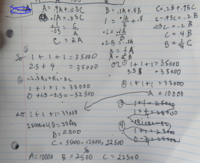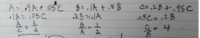# how to set this up

A = 0.9A + 0.05C

B = 0.1A + 0.8B

C = 0.2B + 0.95C

A + B + C = 35k

#### allegansveritatem

##### Full Member
A = 0.9A + 0.05C

B = 0.1A + 0.8B

C = 0.2B + 0.95C

A + B + C = 35k
Thanks for reply. What puzzles me here is how to set up a matrix with these components. This problem comes from the exercises after the section of the text that introduces the use of matrices. All the members of the matrices so far have been monomials. Well, I will copy the expressions here down and see what I can figure out later today.

#### allegansveritatem

##### Full Member
one thing I forgot to add: As yet I have not dealt with matrices with variables in the last far right column.

#### Dr.Peterson

##### Elite Member
Thanks for reply. What puzzles me here is how to set up a matrix with these components. This problem comes from the exercises after the section of the text that introduces the use of matrices. All the members of the matrices so far have been monomials. Well, I will copy the expressions here down and see what I can figure out later today.
one thing I forgot to add: As yet I have not dealt with matrices with variables in the last far right column.
Rewrite each equation in standard form (variables on the left, constants on the right). You have presumably learned how to put that into matrix form. (And the elements of the matrix will be numbers, not monomials.)

#### allegansveritatem

##### Full Member
Rewrite each equation in standard form (variables on the left, constants on the right). You have presumably learned how to put that into matrix form. (And the elements of the matrix will be numbers, not monomials.)
Here is what I did today before I read the above post from Dr Peterson:I don't know if the results are correct but I doubt the means I obtained them are exactly what the author of the exercise had in mind.
I will try what you suggest. I think in the above image you may find something like that was attempted.

#### Jomo

##### Elite Member
Please do not write 1 + 1 + 1 =3500. Please do not write such things.

•JeffM, HallsofIvy and topsquark

#### skeeter

##### Elite Member
$$\displaystyle \begin{bmatrix} 1 & 1 & 1\\ 0.1 &-0.2 & 0\\ 0 & 0.2 & -0.05 \end{bmatrix} \cdot \begin{bmatrix} A\\ B\\ C \end{bmatrix}=\begin{bmatrix} 35\\ 0\\ 0 \end{bmatrix}$$

A = 10k, B = 5k , C = 20k

•topsquark

#### allegansveritatem

##### Full Member
Please do not write 1 + 1 + 1 =3500. Please do not write such things.
Right. I will try to do it the manner prescribed.

#### allegansveritatem

##### Full Member
$$\displaystyle \begin{bmatrix} 1 & 1 & 1\\ 0.1 &-0.2 & 0\\ 0 & 0.2 & -0.05 \end{bmatrix} \cdot \begin{bmatrix} A\\ B\\ C \end{bmatrix}=\begin{bmatrix} 35\\ 0\\ 0 \end{bmatrix}$$

A = 10k, B = 5k , C = 20k
I will have to work through this to make sure I understand it. Thanks.

#### allegansveritatem

##### Full Member
Rewrite each equation in standard form (variables on the left, constants on the right). You have presumably learned how to put that into matrix form. (And the elements of the matrix will be numbers, not monomials.)
I tried to put these into standard form so that I would have the variables on one side and the following is the best I could do:But how to use these in a matrix?

#### Dr.Peterson

##### Elite Member
I tried to put these into standard form so that I would have the variables on one side and the following is the best I could do:
View attachment 22438
But how to use these in a matrix?
I see nothing there that is what I meant by "standard form (variables on the left, constants on the right)".

One example is "x + y + z = 35000".

Another is "0.1A - 0.05C = 0". To get the matrix more directly from the equation, you could write it as "0.1A + 0B - 0.05C = 0".

Perhaps you need to show us what you have learned about how to turn a system of equations into a matrix. I thought this would be the first thing they would teach you.

#### allegansveritatem

##### Full Member
I see nothing there that is what I meant by "standard form (variables on the left, constants on the right)".

One example is "x + y + z = 35000".

Another is "0.1A - 0.05C = 0". To get the matrix more directly from the equation, you could write it as "0.1A + 0B - 0.05C = 0".

Perhaps you need to show us what you have learned about how to turn a system of equations into a matrix. I thought this would be the first thing they would teach you.
Well, now that I look at it...I see what you are getting at. I don't know exactly what I was thinking...I looked at the system of equations that Skeeter suggested and, instead of replacing the A and C with 10000 and 20000 and thus seeing that they were obviously true, I somehow assumed that they had to be impossible. I knew that what I did was not what was asked for but...OK. I will go back now and do it right. Thanks for pointing it out. Sometimes in doing an exercise I get stuck in a loop of error and just don't see the elephant in the room.